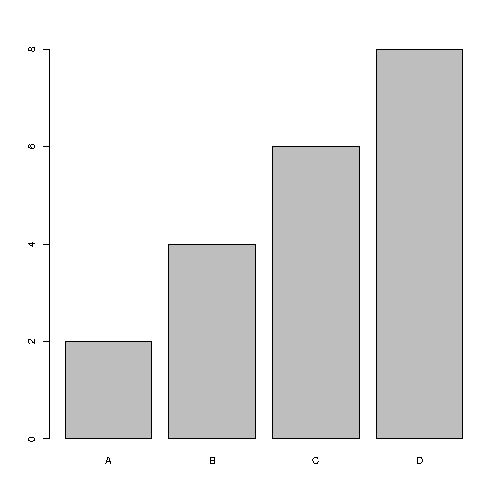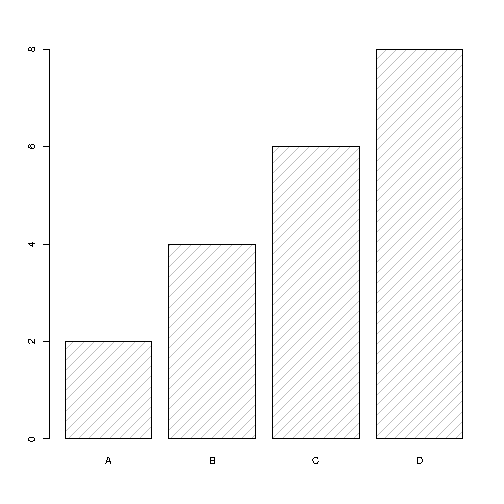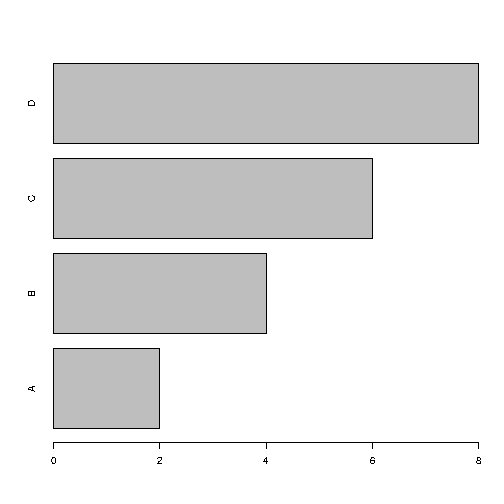# R Bar Charts

## Bar Charts

A bar chart uses rectangular bars to visualize data. Bar charts can be displayed horizontally or vertically. The height or length of the bars are proportional to the values they represent.

Use the `barplot()` function to draw a vertical bar chart:

### Example

# x-axis values
x <- c("A", "B", "C", "D")

# y-axis values
y <- c(2, 4, 6, 8)

barplot(y, names.arg = x)

Result:Try it Yourself »

#### Example Explained

• The `x` variable represents values in the x-axis (A,B,C,D)
• The `y` variable represents values in the y-axis (2,4,6,8)
• Then we use the `barplot()` function to create a bar chart of the values
• `names.arg` defines the names of each observation in the x-axis

## Bar Color

Use the `col` parameter to change the color of the bars:

### Example

x <- c("A", "B", "C", "D")
y <- c(2, 4, 6, 8)

barplot(y, names.arg = x, col = "red")

Result:Try it Yourself »

## Density / Bar Texture

To change the bar texture, use the `density` parameter:

### Example

x <- c("A", "B", "C", "D")
y <- c(2, 4, 6, 8)

barplot(y, names.arg = x, density = 10)

Result:Try it Yourself »

## Bar Width

Use the `width` parameter to change the width of the bars:

### Example

x <- c("A", "B", "C", "D")
y <- c(2, 4, 6, 8)

barplot(y, names.arg = x, width = c(1,2,3,4))

Result:Try it Yourself »

## Horizontal Bars

If you want the bars to be displayed horizontally instead of vertically, use `horiz=TRUE`:

### Example

x <- c("A", "B", "C", "D")
y <- c(2, 4, 6, 8)

barplot(y, names.arg = x, horiz = TRUE)

Result:Try it Yourself »

W3Schools is optimized for learning and training. Examples might be simplified to improve reading and learning. Tutorials, references, and examples are constantly reviewed to avoid errors, but we cannot warrant full correctness of all content. While using W3Schools, you agree to have read and accepted our terms of use, cookie and privacy policy.